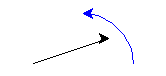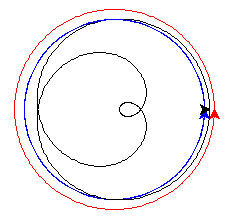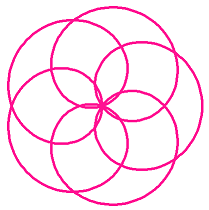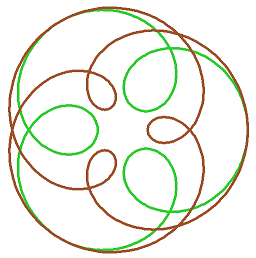# Celestial Wheels¶

This project draws a loopy curve, that mathematicians call an epitrochoid, and everyone else calls a spirograph pattern. It introduces the idea of an object and its methods.

## Turtles, turtles, turtles¶

In earlier projects we used only one turtle, with no name, and your commands like `forward` and `left`, went to that unnamed turtle. Here you’ll create two other turtles, and send commands to each of them by name.

First, let’s see how this works. Start the project with an empty Trinket. Type in the editor:

```# Epitrochoids
from turtle import *

# Test
ted = Turtle()
```

This is how you make a new turtle, and a variable `ted` to refer to it. Now we can give `ted` the turtle some things to do. Add and run this:

```ted.color("blue")
ted.left(60)
ted.forward(100)
```

These instructions work like function calls, but addressed to `ted`. A function addressed to a particular object is called a method. The dot `.` is how you address a method to an object in Python. It has to be something the object knows how to do, so you can’t say `ted.print()`, because turtles don’t know that word. The unnamed turtle is still there and waiting for your instructions:

```forward(100)
```

Delete the test code so your Trinket looks like this again:

```# Epitrochoids
from turtle import *

# Test
```

## Setting up the guide turtles¶

For the next part, we need two “guide” turtles, each of which draws a circle. Each guide has to be set up a certain distance from home, so add these two functions to your code (above `# Test`), so it looks like this:

```# Epitrochoids
from turtle import *

def new_guide(r, c):
"Return a new guide turtle of radius r and colour c"
t = Turtle()
t.speed("fastest")
t.color(c)

# Go to (r,0) without drawing
t.penup()
t.setposition(r,0)
t.left(90)
t.pendown()

return t

def mid(p, q):
"Half way between positions p and q"
x = (p + q) / 2
y = (p + q) / 2
return x, y

# Test
```

Notice that the `new_guide` function returns a turtle. Add this code starting after `# Test` and run the program:

```g = new_guide(100, "blue")
start = g.position()
g.circle(50, 90)
end = g.position()
setposition(mid(start,end))
```

In that last line we did two things at one: the `mid` function calculates the half-way point between `start` and `end`, then `setposition` takes your unnamed turtle there. You should see this:The unnamed turtle (black) has moved halfway between start and end of the blue arc. When that works, delete the test code again.

## Moving the guides¶

The first job is to make the guides move in their orbits. Add this function to your program, and call it as a test:

```def epitrochoid(a, b, L, M=1):
ta = new_guide(a, "blue")
tb = new_guide(b, "red")

# N little steps s make one circle
N = 500
s = 360/N
for i in range(N):
ta.circle(a, L*s)
tb.circle(b, M*s)

# Test
epitrochoid(90, 100, 3, 2)
```

Run that. You should see blue and red circles drawn.

The blue turtle goes round L=3 times, and the red turtle M=2 times. You can see how this works in the code. `N` just has to be a big enough number to make the final curve smooth. `N` steps of size `s` make 360 degrees, exactly one circle. So `N` steps of `L` or `M` steps make `L` or `M` full circles.

`M=1` on the first line says that, if you don’t give it a value in the call to `epitrochoid`, `M` will be equal to 1.

## Compute the shape¶

The shape we are looking for is drawn by keeping our unnamed turte mid-way between the two guide turtles.

Add lines to the `epitrochoid` function so it reads like this:

```def epitrochoid(a, b, L, M=1):
ta = new_guide(a, "blue")
tb = new_guide(b, "red")

# Set start position for unnamed turtle
penup()
setposition((a+b)/2, 0)
pendown()

# N little steps s make one circle
N = 500
s = 360/N
for i in range(N):
ta.circle(a, L*s)
tb.circle(b, M*s)
m = mid(ta.pos(), tb.pos())
setposition(m)
```

Run the code. You should see this:## Tidy up¶

It would be nice if the guide circles were not on the final drawing. Change the function `new_guide`, so guide turtles are invisiible (`hideturtle`) and do not draw (do not `pendown`).

```def new_guide(r, c):
"Return a new guide turtle of radius r and colour c"
t = Turtle()
t.speed("fastest")
t.color(c)

# Go to (r,0) without drawing
t.penup()
t.setposition(r,0)
t.left(90)
t.hideturtle()
#t.pendown()

return t
```

Style the unnamed turtle to your liking:

```# Test
speed("fastest")
width(5)
color("lime green")
epitrochoid(90, 100, 3, 2)

hideturtle()
```

## Inspiring examples¶

Try changing the numbers in the call to `epitrochoid` like this:

```a, b = 50, 150
epitrochoid(a, b, 4)

color("goldenrod")
epitrochoid(a, b, 5)

color("sienna")
epitrochoid(a, b, 6)
```

(Remember, M=1 if you don’t give a fourth argument.) Suppose you change just one line now:

```a, b = -50, 150
```

and run again. When the loops point outwards, the shape is called a hypotrochoid.

What’s happening here?

```a, b = 250, 300
epitrochoid(a, b, 4)

color("goldenrod")
epitrochoid(a, b, 5)

color("sienna")
epitrochoid(a, b, 6)
```

```L = 6
a, x = 20, 50
epitrochoid(a, L*a, L)

color("goldenrod")
epitrochoid(a, L*a + x, L)

color("sienna")
epitrochoid(a, L*a - x, L)
```

Find other interesting shapes of your own.

• What values for `a` and `b` make the curve pass through (0,0)? (Hint: where would the guide turtles be at that moment?)